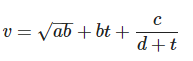# dimensional analysis practice problems

In this page we have dimensional analysis practice problems . Hope you like them and do not forget to like , social share and comment at the end of the page.

Question 1
The air bubble formed by explosion inside water perform oscillations with time period T which depends on pressure (p), density (ρ) and on energy due to explosion (E). Establish relation between T, p, E and ρ.

Question 2
The velocity v of a particle depends upon the time ‘t’ according to the equationDetermine the units of a, b, c and d. What physical quantities they represent. All have SI units.

Question 3
Mass of the Sun is $2.0 \times 10^{30}$ Kg and radius of sun is $7.0 \times 10^{8}$m. Express the density of sun. Also explain why the order of magnitude of density of sun is in the range of solids and not in the range of liquids and gases?

Question 4
A physical quantity X is connected from $X=\frac{a{{b}^{2}}}{C}$ . Calculate the percentage error in X, when % error in a, b, c are 4, 2, and 3 respectively.

Question 5
Percentage error in the measurement of height and radius of cylinder are $x$ and $y$ respectively. Find the percentage error in the measurement of volume. Which of the two measurements height or radius needs more attention?

Question 6
Orbital radius of mercury around the sun is 0.38 A.U. Find the angle of maximum elongation for mercury and its distance from earth when the elongation is maximum.

Question 7
Convert 4.29 light years into parsecs. Calculate the parallax of a star at this distance when viewed from two locations of earth six months apart in its orbit around the sun.

Question 8
In an experiment, on determining the density of a rectangular block, the dimensions of the block are measured with a Vernier calipers (with a least count of 0.01 cm) and its mass is measured with a beam balance of least count of 0.1 g. The measured values are Lenght(L) = 5.12 cm, Breadth(b) = 2.56 cm, thickness(t) = 0.37 cm and mass(m) = 39.39 g.How do we report our result for the density of the block.

Question 9
It is required to find the volume of a rectangular block. A Vernier callipers is used to measure the length, width and height of the block. The measured values are 1.37 cm, 4.11 cm, and 2.56 cm respectively.

Question 10
Two specific heat capacities of a gas are measured as Cp=(12.28±0.2) units and CV=(3.97±0.3) units. Find the value of gas constant R.

### Theory questions on error analysis

Note:- Write all the points you know about these questions when you write the answers.
Question 1
Define error. What are different sources of errors?
Question 2
How can we minimize errors?
Question 3
What are the different ways of expressing an error?
Question 4
What is Absolute Error? Define the term accuracy.

Question 5
What do you mean by precision? According to you are instrument of high precision accurate?

Question 6
What is relative error or percentage error?

Question 7
What is Parallax error?

Question 8 How errors are propagated or combined?